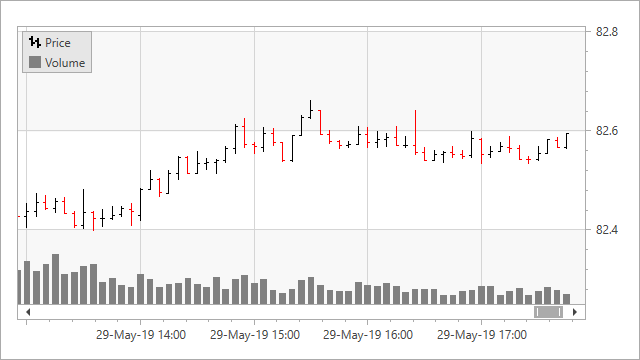21.1
20.2
20.1
19.2
19.1
18.2
18.1
17.2
A newer version of this page is available. Switch to the current version.

# Data Aggregation

• 6 minutes to read

The Chart Control can use an aggregate function to group raw data source values and show aggregates as series points. You can use aggregation to show grouped data and decrease the number of visible points in a chart. Data aggregation is only available for argument axes (x-axes).## Aggregation vs Summaries

In addition to Aggregation, the Chart Control provides Summaries that also allow you to group raw data values.

Aggregation Summary
Store Data Chart stores raw data source values. Chart stores summarized values.
Change Detail Level Chart re-calculates data in memory. Chart re-loads data from the data source.
Behave on Zoom If the automatic scale options are used, Chart recalculates value aggregates when it is zoomed. Chart does not re-calculate aggregated values when it is zoomed.
Memory Consumption High Low

## Aggregation Basics

When the aggregation is enabled, the chart splits the x-axis into intervals (the x-axis measurement unit value defines the intervals), and uses an aggregate function to aggregate data for each interval.

The AggregateFunction enumeration lists the available aggregate functions:

• Average (a default function)
• Count
• Financial (Use it for financial series.)
• Histogram (See the Histogram topic for more information.)
• Maximum
• Minimum
• Sum
• Custom (Refer to the Create an Aggregate Function section for more information.)

Scale options assigned to the x-axis’s ~ScaleOptions property store aggregation-related settings. You can use the following option types:

Options

Description

How to use

Automatic Date-Time and Automatic Numeric

The chart control defines the optimal measurement unit for an axis based on the data set’s values, the Chart Control’s current size, and zoom level.

For date-time axes. Assign a AutomaticDateTimeScaleOptions object to the AxisX2D.DateTimeScaleOptions, AxisX3D.DateTimeScaleOptions or RadarAxisX2D.DateTimeScaleOptions property.
For numeric axes. Assign a AutomaticNumericScaleOptions object to the AxisX2D.NumericScaleOptions, AxisX3D.NumericScaleOptions or CircularAxisX2D.NumericScaleOptions property.

Manual Date-Time and Manual Numeric

You can specify the axis measurement unit.

For date-time axes. Assign a ManualDateTimeScaleOptions (or its descendant) object to the AxisX2D.DateTimeScaleOptions, AxisX3D.DateTimeScaleOptions or RadarAxisX2D.DateTimeScaleOptions property.
For numeric axes. Assign a ManualNumericScaleOptions (or its descendant) object to the AxisX2D.NumericScaleOptions, AxisX3D.NumericScaleOptions or CircularAxisX2D.NumericScaleOptions property.

Qualitative

Chart aggregates point values if points have equal arguments.

For qualitative axes. Assign QualitativeScaleOptions to the AxisX2D.QualitativeScaleOptions or AxisX3D.QualitativeScaleOptions property.

##### NOTE

Axes that have the Continuous scale type (ContinuousDateTimeScaleOptions and ContinuousNumericScaleOptions) do not support aggregation.

## Aggregate Date-Time Data

The following images show aggregated date-time data:

The examples below use an Average function to aggregate date-time data:

### Automatic Mode

``````<dxc:XYDiagram2D>
<dxc:XYDiagram2D.AxisX>
<dxc:AxisX2D>
<dxc:AxisX2D.DateTimeScaleOptions>
<dxc:AutomaticDateTimeScaleOptions AggregateFunction="Average"/>
</dxc:AxisX2D.DateTimeScaleOptions>
</dxc:AxisX2D>
</dxc:XYDiagram2D.AxisX>
<!--...-->
</dxc:XYDiagram2D>
``````

### Manual Mode

``````<dxc:XYDiagram2D>
<dxc:XYDiagram2D.AxisX>
<dxc:AxisX2D>
<dxc:AxisX2D.DateTimeScaleOptions>
<dxc:ManualDateTimeScaleOptions AggregateFunction="Average"
MeasureUnit="Year"
GridAlignment="Year"/>
</dxc:AxisX2D.DateTimeScaleOptions>
</dxc:AxisX2D>
</dxc:XYDiagram2D.AxisX>
<!--...-->
</dxc:XYDiagram2D>
``````

The examples above use the following API members:

Member Description
AxisX2D.DateTimeScaleOptions Provides access to the options that define the behavior of a date-time X-scale when its mode is manual, automatic or continuous.
AutomaticDateTimeScaleOptions Contains settings for a date-time axis data when its scale mode is automatic.
ManualDateTimeScaleOptions Contains settings for a date-time axis data when its scale mode is manual.

### Form a Custom Measurement Unit

You can use the ManualDateTimeScaleOptions.MeasureUnitMultiplier property with ManualDateTimeScaleOptions.MeasureUnit to specify a new measurement unit.

``````<dxc:XYDiagram2D>
<dxc:XYDiagram2D.AxisX>
<dxc:AxisX2D>
<dxc:AxisX2D.DateTimeScaleOptions>
<dxc:ManualDateTimeScaleOptions AggregateFunction="Average"
GridAlignment="Year"
MeasureUnit="Month"
MeasureUnitMultiplier="6"/>
</dxc:AxisX2D.DateTimeScaleOptions>
</dxc:AxisX2D>
</dxc:XYDiagram2D.AxisX>
</dxc:XYDiagram2D>
``````

See also the following examples:

## Aggregate Numeric Data

The following images show aggregated numeric data:

The examples below use an Average function to aggregate numeric data:

### Automatic Mode

``````<dxc:XYDiagram2D>
<dxc:XYDiagram2D.AxisX>
<dxc:AxisX2D>
<dxc:AxisX2D.NumericScaleOptions>
<dxc:AutomaticNumericScaleOptions AggregateFunction="Average"/>
</dxc:AxisX2D.NumericScaleOptions>
</dxc:AxisX2D>
</dxc:XYDiagram2D.AxisX>
</dxc:XYDiagram2D>
``````

### Manual Mode

``````<dxc:XYDiagram2D>
<dxc:XYDiagram2D.AxisX>
<dxc:AxisX2D>
<dxc:AxisX2D.NumericScaleOptions>
<dxc:ManualNumericScaleOptions AggregateFunction="Average"
MeasureUnit="1000"/>
</dxc:AxisX2D.NumericScaleOptions>
</dxc:AxisX2D>
</dxc:XYDiagram2D.AxisX>
</dxc:XYDiagram2D>
``````

The examples above use the following API members:

Member Description
AxisX2D.NumericScaleOptions Provides access to the options that define the behavior of a numeric X-scale when its mode is manual, automatic or continuous.
AutomaticNumericScaleOptions Contains settings for a numeric axis data when its scale mode is automatic.
ManualNumericScaleOptions Contains settings for a numeric axis data when its scale mode is manual.

See also the following examples:

## Aggregate Qualitative Data

The following images show non-aggregated and aggregated qualitative data:

The example below uses a Sum function to aggregate qualitative data:

``````<dxc:XYDiagram2D>
<dxc:XYDiagram2D.AxisX>
<dxc:AxisX2D>
<dxc:AxisX2D.QualitativeScaleOptions>
<dxc:QualitativeScaleOptions AggregateFunction="Sum"/>
</dxc:AxisX2D.QualitativeScaleOptions>
</dxc:AxisX2D>
</dxc:XYDiagram2D.AxisX>
</dxc:XYDiagram2D>
``````

## Aggregate an Individual Series’s Data

You can apply different aggregate functions to series that are bound to the same x-axis. In this case, the aggregate function applied to a series has higher priority than the axis’s aggregate function. To specify a series’s aggregate function, use the XYSeries.AggregateFunction property.The following example uses the Financial function to aggregate the Stock series and the Sum function - the Bar series:

``````<dxc:XYDiagram2D>
<dxc:StockSeries2D
x:Name="stockSeries"
DisplayName="Price"
ArgumentDataMember="DateTimeStamp"
OpenValueDataMember="Open"
HighValueDataMember="High"
LowValueDataMember="Low"
CloseValueDataMember="Close"
AggregateFunction="Financial">
</dxc:StockSeries2D>
<dxc:BarSideBySideSeries2D
x:Name="volumeSeries"
DisplayName="Volume"
ArgumentDataMember="DateTimeStamp"
ValueDataMember="Volume"
AggregateFunction="Sum">
</dxc:BarSideBySideSeries2D>
</dxc:XYDiagram2D>
``````

## Create a Custom Aggregate Function

The example below shows how to implement an aggregate function:

``````<dxc:XYDiagram2D>
<dxc:XYDiagram2D.AxisX>
<dxc:AxisX2D>
<dxc:AxisX2D.DateTimeScaleOptions>
<dxc:AutomaticDateTimeScaleOptions AggregateFunction="Custom">
<dxc:AutomaticDateTimeScaleOptions.CustomAggregateFunction>
<local:StandardDeviationAggregateFunction/>
</dxc:AutomaticDateTimeScaleOptions.CustomAggregateFunction>
</dxc:AutomaticDateTimeScaleOptions>
</dxc:AxisX2D.DateTimeScaleOptions>
<!--...-->
</dxc:AxisX2D>
</dxc:XYDiagram2D.AxisX>
<!--...-->
</dxc:XYDiagram2D>
``````